# The Development of Algebra - 2

##### Age 11 to 18

Published 2011

The first part of this brief history of algebra focussed on the important practical origins of the problems that led to the procedures we have for solving equations, and the ways in which the problems were visualised as manipulation of geometrical shapes.

This second part shows how the visual images slowly give way to literal representations, abbreviations, and finally, in the 17th century, to a more developed algebraic symbolism close to what we use in schools today [see: Note 1].

### 1. Mediaeval Algebra

The expansion of the Arab Empire into Asia Minor (Modern Turkey) at the end of the 11th century led to a series of Crusades to recapture the Holy Land. The major period of these military and political disturbances lasted until the end of the 14th century. In 1340 the 'Black Death' spread into Western Europe killing some 40% of the population by about 1370. In spite of these upheavals, exchange of ideas and translations of Arab scholarship were brought to Europe. After 1450 the printing press enabled many people to read the Latin translations of Arab and Greek science.

Mediaeval Algebra in Western Europe was first learnt from the works of al-Khowarizmi, Abu Kamil and Fibonacci. The algebra consisted of simple linear and quadratic equations and a few cubic equations, together with the methods for solving them; rules for operating with positive and negative numbers, finding squares, cubes and their roots; the rule of False Position (see History of Algebra Part 1 ) and the Rule of Three (simple proportion). These methods were applied to business and legal problems. There was some justification of the solution using diagrams, but mostly it was a matter of 'memorising the rules' and applying them to standard problems.

Leonardo Fibonacci (1170 - 1250)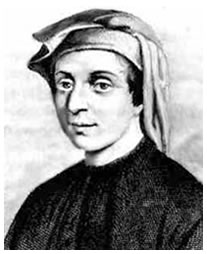Well known for his collection of mathematical techniques [see: Note 2] and the promotion of the Hindu numeral system in the Liber Abaci of 1202, he also wrote Flos , a book where he shows that the root of the cubic equation $10x + 2x^2 + x^3 = 20$ can neither be a rational number, nor the square root of a rational number [see: Note 3].

In his Book of Squares 1225 he turned much of Euclid's geometrical work into arithmetic, developing new ideas from Arab science, and other techniques used by the 'Abacus Masters' who taught commercial arithmetic [see: Note 4]. He organised the rules as a series of logical propositions and supported his arguments using proportional triangles, squares and rectangles, which as we have seen, are perfectly general.

Proposition 1 shows how the sum of the odd numbers always makes a perfect square. In our notation, the substance of Leonardo's argument is:$$(1+3+5+7)+9=(1+3+5+7+9)$$

Proposition 2 states "Any square number exceeds the square before it by the sum of the roots." Leonardo's first example is simple:
$$5^2 - 4^2 = 25 - 16 \mbox{, which is } 9 \mbox {, the sum of } 5 \mbox { and } 4\mbox {, which are the roots of }25 \mbox{ and } 16.$$
Proposition 10 finds the sum of a sequence of square numbers: $$6(1^2 + 2^2 + 3^2 +.... + n^2) = n(n + 1)( 2n + 1)$$
Proposition 19 shows how to find numbers $A$, $B$, and $C$ such that: $$B^2 - B = A^2 \mbox { and } B^2 + B = C^2$$

These and many other numerical relationships helped mathematicians of the 15th and 16th centuries to develop techniques for solving quadratic and cubic equations.

Jordanus de Nemore (1225 - 1260)
The mediaeval student's attitude towards solving equations was quite different to ours. Today we arrange the algebra to isolate the unknown and we make $x$ the 'subject' of the equation. In Mediaeval times, the student worked with the known numbers in order to find the 'root'. Jordanus' book, De Numeris Datis (Concerning given numbers) was written about 1250 and is considered to be the first advanced algebra written in Western Europe since Diophantos . It offers a generalised treatment of quadratic, simultaneous and proportional equations. For solving quadratics, the procedure earlier described by Abu Kamil (c850 - c930) as 'completing the square' was simplified, Part 1 (Section 7 Case 5) , and for a problem like 'A square and 10 of its roots equal 39' you make a square, and attach to two of its sides rectangles whose length is half the number of roots, and proceed to fill in the empty space with another square.

####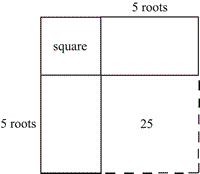##### In the diagram, since the rectangles are $5$ roots long the dotted square must be $25$ roots. But the total of square and roots are given as $39$, so the side of the unknown square must be $3$, and the whole square $64$.

The 'Datis ' shows how, by analysis of basic geometrical properties and using letters of the alphabet to represent numerical relationships, it is possible to establish generalised knowledge. Jordanus then illustrates each case with numerical examples.

For example, in Book IV Proposition 6, he shows that "If the ratio of two numbers and the sum of their squares is known, then each of the numbers is known." [see: Note 6].
In modern symbols, he proceeds like this:$$\mbox{Given, }x : y = a \mbox { and } x^2 + y^2 = b$$

Let $d$ be the square of $x$, and $c$ the square of $y$, and let $d + c$ be known , so we have:
$$x : y = a, ~~~~~~~x^2 = d, ~~~~~~~y^2 = c, ~~\mbox {and so } ~~d + c = b$$
Now the ratio of $d$ to $c$ is the square of the ratio of $x$ to $y$, so $$\frac{d}{c}=\frac{x^2}{y^2}=a^2 ~~~~~~~~\frac{d}{c}y^2=b-y^2 ~~~~~~~~ \left(\frac{d}{c}+1\right)y^2=b~~~~~~~~ \mbox {and so }y=\sqrt{\frac{b}{\left(a^2+1\right)}}$$
$$\text{For example, if }\frac{x}{y}=2 \mbox { and } x^2+y^2=500 \mbox { then }y=\sqrt{\frac{500}{\left(4+1\right)}} = 10\mbox {, and }x=20 ~~~~~~~~~~~~~~~$$

The work of Jordanus was a significant change in the way equations were tackled. Much more emphasis was given to the number relationships that were based on proportional reasoning. It was the understanding of these relationships that was so important to Francois Viete later in the sixteenth century.

### 2. Early Renaissance Algebra

Nicolas Chuquet (1445 - 1488)
Nicolas Chuquet was described as an 'algoriste' and his manuscript on Le Triparty en la Science des Nombres (1484) was known only to a few of his contemporaries. 'Triparty' means three parts, and the first section was on Numbers and their operations; the second on Surds $\left(3+\sqrt{5}\right)$ and Roots of Surds $\sqrt{\left(3+\sqrt{5}\right)}$; and the third on Algebra, where he invented special symbols for the unknown, squares and cubes up to the fourth power, and a system of indices which included $x^0 = 1$. He also used the first letters of operations like p for plus and m for minus. His algebra was developed as a series of general methods and in this work negative numbers appear as coefficients, exponents and solutions to problems. His rules for solving arithmetic problems also used zero and negative numbers. Unfortunately his work was little known, and not published until 1880, but his ideas reappear in the early 17th century.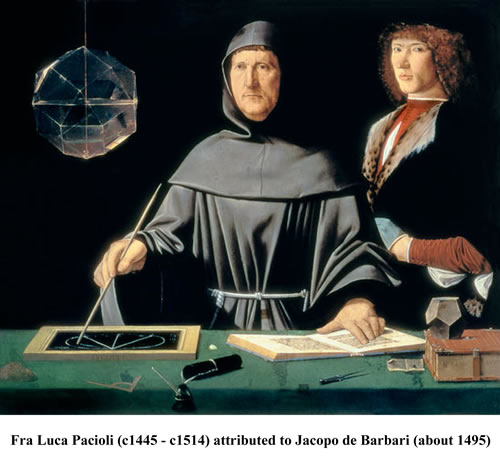Luca Pacioli (1445 - 1517)
Pacioli was a contemporary of Chuquet, and famous for the Summa de Arithmetica, geometria, proportioni et proportionibus (1494) and the Divina Proportione (1509) Pacioli's works were important popular collections of current practical mathematics and were more useful for passing on known techniques and problems than for any original contributions. Pacioli is also famous for publishing the first description of double entry book-keeping where negative numbers had an obvious practical significance as debts or losses, and numerous works on accounting soon appeared in other languages, obviously copied from Pacioli [see: Note 7].

### 3. Late Renaissance and Early Modern Algebra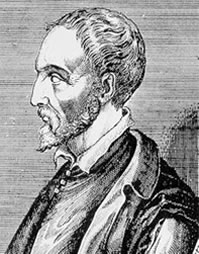Girolamo Cardano (1501 - 1576)
Cardano earned his living as a doctor and by casting horoscopes; he wrote on probability and published other books but his importance for us rests on his Artis Magnae sive de Regulis Algebraicis Liber Unus (1545) "Of the Great Art, or the First Book on the Rules of Algebra" the 'Ars Magna' as it is often called [see: Note 8].

While the methods for solving quadratic problems were well known as a collection of geometrically based proportional relations and arithmetical algorithms, a unified and general approach was still not commonly available.

The big unsolved problem of the time was finding solutions to cubic equations. By this time mathematicians had identified about 13 different cases of cubic equations which included various combinations of cubes, squares and numbers [see: Note 9]. The Ars Magna contains the proportional methods and rules that had been developed by mathematicians before him, his own work was also a significant contribution, and he acknowledged the discoveries of his contemporaries.

Existing methods for solving cubic equations relied on finding substitutions to reduce them to quadratics; many of these tricks could only be applied to special cases. For example this is the first problem in Chapter XXV:

When the cube is equal to the first power and a constant, divide the coefficient of x into two parts that the sum of each multiplied by the square root of the other is half the constant of the equation. The roots of these two parts added together make the value of $x$.
Example: $x^3 = 10x + 24$

Solution: "Ten divides into two parts, $9$ and $1$, either of which multiplied by the square root of the other makes $9$ and $3$, the sum of which is $12$, one half of $24$. Therefore R $9$ plus R $1$ which are $3$ and $1$ added together, produce $4$, the value of x."[see: Note 10]

Throughout the book, every rule was written in Latin; the only notations used were p for plus and m for minus and an elaborate capital R for radix, to indicate square roots.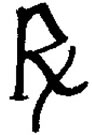$~~~~~$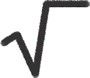### Discovering Imaginary Numbers

In Chapter XXXVII Cardano discusses the use of negative numbers in calculations, and in Rule II appears his first use of negative square roots.
The problem is to 'divide $10$ into two parts whose product is $40$'
His method is exactly the same as the 'Babylonian Algorithm' shown in Part 1 (Teachers' notes 5) and if we think of the problem as: $x(10 - x) = 40$, we have a quadratic equation $x^2 + 40 = 10x$ (squares and numbers equal roots) with $10$ as the coefficient of $x$ which we divide in half, and proceed with the algorithm .

Cardano gives two solutions
$5$p : R : m $15$ and $5$m : R : m $15~~$. [see: Note 11]

The product of these two results is, in fact $40$, and he demonstrates how to solve four other problems that give negative square roots. He was clearly mystified by these 'imaginary' square roots of negative numbers, and this is still a problem for many who meet them for the first time.

### 4. Introducing the 17th Century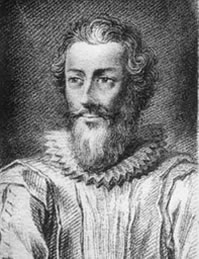Francois Viete (1540-1603)
In his two major works, In Artem Analyticem Isagoge (Introduction to the Analytic Art, 1591) and De Potestatum Resolutione (On the Numerical Resolution of Powers 1600) Viete made probably the most important contributions to the development of algebra at this time.

Viete used a consistent symbolic notation with vowels A, E, I, O, U and Y for unknown quantities, and given terms by the letters B, G, D and other consonants.

Addition and Subtraction used the symbols + and -. For Multiplication he used the word 'in' and for Division used the fraction bar. So $$\frac{\mbox {B in C}}{\mbox {AQ}} \mbox { meant } \frac{\mbox {BC}}{A^2}$$
Powers were N (for numerus - a pure number), Q (quadratus - a square) C (cubus - a cube) further powers were expressed by combinations of these symbols, so QQ was a fourth power, CQ, a fifth power and so on.

For Roots he used the symbol $L$ (for Latus - a side) and sometimes the $R$ symbol . So $L9$ meant the square root of $9$, and $LC 27$ meant the cube root of $27$.
All his equations were homogeneous - that means the dimensions of all the terms in the equation had to be the same.
In the equation $~~~~AQ + AB = Z Q, ~~~~~AQ$ and $ZQ$ represent squares, and AB a rectangle.

Some of these notational ideas were already being used; for example, Johannes Scheubel (1494 - 1570) writing in 1551 used special combinations of symbols for powers of the unknown: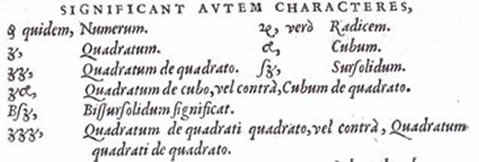The English mathematician, Robert Recorde used the same notation in his book [see: Note 12] on algebra in 1557 but describes the powers of numbers in this way: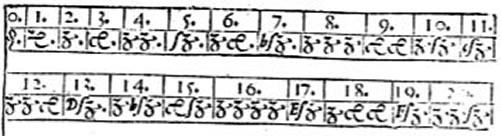Using his literal notation, Viete exposed the structural aspects of polynomial equations and gave solution methods for whole classes of equations.
Viete's solution of quadratic equations used the three proportional triangles in a semi-circle shown in Part 1 (Section 6. Greek Geometry)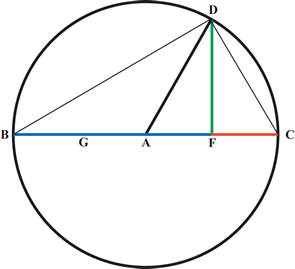The equation: $A$ quadratus $+ AB = Z$ quadratus or $(A^2 + AB = Z^2)$, in Viete's symbols is $AQ + AB = Z Q$, which can be written as $A(A+B)=ZZ$ and rearranged as equal proportions becomes: $$\frac{A}{Z}=\frac{Z}{(A+B)}$$

In the diagram there are three lines of increasing magnitude, $FC, FD$ and $FB$.
$FC$ is $A$, $~~~~~FD$ is $Z~~$ and $FB$ is $A+B$
For three magnitudes in proportion, the well-known rule is:
'the product of the extremes is equal to the square of the mean'.
The lines $A$ and $(A+B)$ are the extremes and $Z$ is the mean .
For the equation $A^2 + 10A = 144, ~~~~~Z = 12 ~~~$ and $~~~A : 12 = 12 : (A+10)$ so we have to find a number $A$, so that the ratios are equal. The three numbers are $8, 12$ and $18$.

### The Fundamental Theorem of Algebra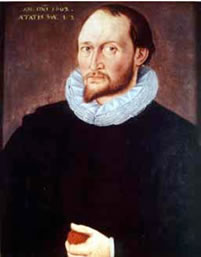Thomas Harriot (c1560 - 1621) and Albert Girard (1595-1632)
By this time, many mathematicians were working on similar problems and developing their own notations. The most important of these were Thomas Harriot, an English mathematician and explorer, and Albert Girard, a Dutch Army Engineer. Harriot's work remains mainly in manuscript form, even today. His Artis Analyticae Praxis (The Practice of the Analytical Art) only appeared in 1661 well after his death, but his papers show that he had developed a sophisticated notation, almost like we use today, using $aa$ for $a^2, aaa$ for $a^3$ etc. and through this realised that multiplying brackets like $(b - a)(c - a)(d - a)$ led to a clear relationship between the roots and coefficients of an equation.

The first explicit statement of the idea that every polynomial equation of degree $n$ has $n$ roots appeared in 1629 in Girard's L'Invention Nouvelle en L'Algebre (A New Discovery in Algebra). Here, Girard states his basic theorem,

"Every algebraic equation .... admits of as many solutions as the denomination of the highest quantity indicates ..."

Girard gave examples, but did not show how he derived his theorem, and did not clearly account for 'imaginary' roots. The search for a general proof occupied mathematicians for many years to come.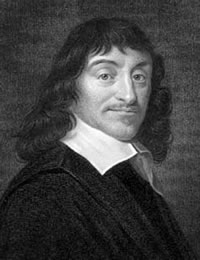Rene Descartes (1596 - 1650)
Even though many improvements in notation had begun to expose the structure of algebraic equations, and begun to transform the representation of the problems from geometric images to written expressions, mathematicians still used geometry as a way of demonstrating the 'truth' and generality of the algebra.

In 1637 he published his Discours de la Methode'... a work on 'how to undertake investigation to make ideas clear, and to seek for truth in the sciences'. As an example of this method he included La Geometrie which states on the first page that,

"Any problem in geometry can easily be reduced to such terms that a knowledge of the length of certain straight lines is sufficient for its construction."

Descartes demonstrates how this may be done: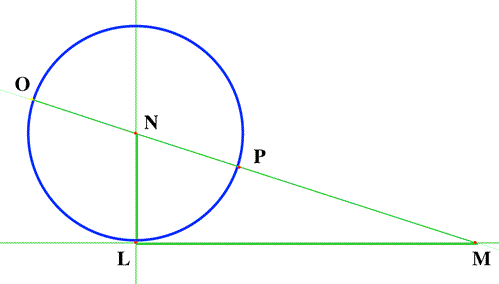The equation is $z^2 \varpropto az-bb$. Construct $LM$ and $LN$ so that $LM =b$ and $LN = \frac{a}{2}$
The unknown $z$, is $OM$. In triangle$NLM$, $$NM^2 =\left(\frac{a}{2} \right)^2 + bb ~~\mbox { and }~~NM = \sqrt{\left(\frac{a}{2} \right)^2 + bb}~~~ \mbox{. So } z=\frac{a}{2}+\sqrt{\left(\frac{a}{2} \right)^2 + bb}.$$
However, Descartes still appealed to a theorem from Euclid Book II Proposition 6 to justify his solution to the reader.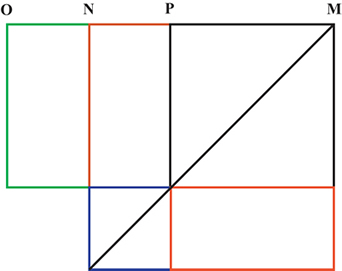The rectangle contained by $OM$ and $PM$, plus the square on $NP$, is equal in area to the square on $NM$. ($N$ bisects $OP$.) The area of the green rectangle is the same as the areas of the red rectangles.
$$OM.PM + NP^2 = NM^2$$
Here is Descartes' original description of the problem: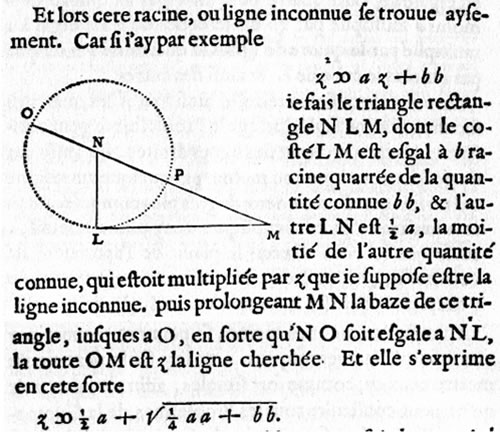### 5. Notation and Representation [see: Note 13]

By the middle of the 17th Century the representation of elementary algebraic problems and relations looked much as it is today. The major factors influencing change were the printing press that provided wider communication of ideas, and the slow appreciation of the similarity in the structure of the algorithms brought about by the changes in notation. Most of this happened in the period from 1500 to 1650; by then the standard notation had become generally accepted.

There were no clear stages in this process. Some historians proposed a 'literal' stage where all the problems are written, sometimes in very complicated language; a 'syncopated' stage with a mixture of words and symbols; and a final 'symbolic' stage where the mathematics consists only of symbols. But this is not the case, even today when you look at a text, words are still there. Another aspect was the technical language . Translating from Greek and Arabic into Latin, and then into the common language of a country, new words were invented to describe new ideas, and were then taken over by others. For example, the German for 'thing' or the unknown, was 'Die Coss', the title of a book by Michael Stifel (1487 - 1567). So, in England, algebra became known as the Cossic Art . Borrowing from the French, the pentagon was known as a cinqangle and so on. There are many more examples among the writings of English mathematicians of the 16th and 17th centuries.

Representation of the objects, relationships, operations, and the structure of processes together with the evolution of the printing press, were the most important aspects which aided the development of mathematics during this period.

The signs for Addition and Subtraction first appear in print in 1526, and the Equals sign appears in 1557. The cross X for Multiplication is later, about 1628; the Colon (: ) for Division in 1633 and the Obelus ($\div$) in 1659. The signs for inequality , > and < are first used in 1631.

Square and other roots start as the capital R (as in the picture above) in about 1465 and eventually our usual sign $\sqrt{}$ by 1630; Powers were first expressed by whole number indices in 1484 and had become widely accepted by 1637, and negative indices also appear in 1484, but fractional indices not until 1676.

The symbols for the unknown and for constants are greatly varied. All kinds of signs and combinations of signs were initially used. Jordanus used letters to replace numbers as a sign of generality, Vieta was the first to use vowels (A, E, I, O, U) for the unknown and consonants (A, B, C, ...) for known quantities, and Descartes introduced the convention of letters at the end of the alphabet (x, y, z) for unknown and at the beginning (a, b, c) for known quantities which is what we use today.

N.B. Teachers' notes related to the history of algebra discussed here can be found by clicking on the 'Notes' tab at the top of this article .

### Notes

1. It is important to note that there were many people throughout this period from the 11th to the 17th century who made significant contributions to the story of the development of algebra, and have not been mentioned here. Further information can be found by consulting the references.
2. We are very lucky that copies of Fibonacci's books have survived. The Liber Abaci (1202 ), Practica Geometriae (1220), Flos and the Book of Squares both produced in 1225 tell us a great deal about mathematics in the early Mediaeval period.
3. Fibonnaci's approximate result is correct to nine decimal places. This equation was solved by Omar Khayyam (1048 - 1122) using the intersection of a circle with a hyperbola.
4. The Abacus Schools were training courses for merchants in commercially useful arithmetic, but they also included 'puzzle problems'. Fibonacci has been mistakenly seen as the father of the Abacus Schools, but they existed well before his time.
5. The Arithmetica of Diophantos (c200 - c284) had been translated and developed by the Arabs and was available in Latin at this time.
6. I have taken this example from the translation by Barnabas Hughes, pages 167-168.
7. It is interesting to contrast the social circumstances and the quality of the work of Chuquet and Pacioli. Chuquet had access to many mathematical works, and made the most of his opportunities in developing original ideas. However he was relatively isolated, hardly went outside his home city of Lyon, and his manuscript work was not printed at the time. Pacioli on the other hand was born into a commercial milleu in Italy, was known to two famous artists, Piero della Francesca and Leone Battista Alberti, was a tutor to the sons of powerful people in Venice and Rome, and had his books printed. This is not to denigrate Pacioli, but only to point out that different circumstances and the printing press played a large part in their fortunes.
8. Most recent histories of mathematics give versions of the story of the solution of equations in 15th and 16th century Italy. Chapter 4 of John Derbyshire's Unknown Quantity gives a good account of the convolutions surrounding Cardan's work.
9. We know that a cubic equation has three roots that are real or imaginary according to whether the graph cuts the x-axis in three places, touches the axis at a minimum, or cuts it only once. Clearly, these concepts were not available to Cardano.
10. The substitution 'trick' comes from the works of Fibonacci and Jordanus: in our notation, if $x^3=ax+N$, let $a=f+g$ and let $f \sqrt{g}+g\sqrt{f}=\frac{N}{2}$ then $x=\sqrt{f}+\sqrt{g}$
11. $5+\sqrt{-15}$ and $5-\sqrt{-15}$
12. Robert Recorde The Whetstone of Witte 1557. Another interesting aspect of the evolution of mathematical understanding is the development of a universal technical language. In England, many words were taken over from French (moitie meaning half) and German (zenzike meaning square). This is where the strange z symbol comes from.
13. For websites with more detailed information on the development of notation see the list of References.

### References

a) General sources covering the mathematics of the Middle Ages and Renaissance:

Boyer, C. B. (1968) A History of Mathematics . London. John Wiley. A popular book with many reprints. Chapters XV and XVI cover the Middle Ages and the Renaissance.

Cajori, F. A. (2007) A History of Mathematical Notations 2 Vols. . This is the principal source book for information in this area. Originally published in 1928/9 by Open Court, there is a new paperback edition available from Amazon at £ 17 for each volume. A bargain for your college library.

Derbyshire, J. (2008) The Unknown Quantity . London. Atlantic Books Now in paperback at £9.99 this is a popular story of the problem of finding the 'thing' of ancient algebra up to the 20th Century. The Introduction and Part 1 up to page 94 1591cover the ideas in both parts of this NRICH account. There are useful sections giving reasonable straightforward explanations of the mathematics involved.

Katz, Victor, J. (1999) (Second Edition, Corrected) A History of Mathematics : An Introduction Harlow, England. Addison-Wesley The best and most comprehensive and up-to-date general history of mathematics available. Chapter 9 'Algebra in the Renaissance' (pages 342 - 384) covers most of the material in this article.

Kline, M. (1972) Mathematical Thought from Ancient to Modern Times. Oxford. O.U.P. Before Katz, this was the best available and has been reprinted a number of times. Chapters 11 to 13 on the Renaissance and its mathematics are still very useful.

b) More specialised sources available in translation:

Girolamo Cardano (1545) The Great Art, or the Rules of Algebra. (Translated by R. Witmer) 1968. M.I.T. Press.

Nicolas Chuquet, Renaissance Mathematician (1985) Translated by Graham Flegg, Cynthia Hay and Barbara Moss of Le Triparty en la Science des Nombres . Lancaster Reidel Publishing Company.

Rene Descartes (1637) La Geometrie (Translated by D.E. Smith and Marcia Latham) 1954. This Dover edition of the Geometry is still available. The English translation is on one page, and a facsimile of the original French on the facing page. The French is not too difficult, and the notation for a quadratic equation virtually the same as today.

Leonardo Fibonacci The Book of Squares (Translated by L.E. Sigler ) 1987) London. Academic Press.

Jordanus de Nemore De Numeris Datis (Translated by Barnabas Hughes) 1981 University of California Press.

Recorde, Robert (1557) The Whetstone of Witte From the original English Text.

Johannes Scheubel (1551) Algebrae Compendiosa From the Original Latin Text Francois

Viete (1591) The Analytic Art (Translated by R. Witmer) 1983 Kent State University Press

The 'MACTUTOR ' is the most comprehensive easily navigable website for the History of Mathematics.
http://www-history.mcs.st-and.ac.uk/history/
Here you can find the biographies of the mathematicians mentioned in this article, including some detail of the relevant mathematics involved.

The earliest use of various mathematical symbols can be found at:
http://jeff560.tripod.com/mathsym.html

Mathematical symbols:
http://nrich.maths.org/public/viewer.php?obj_id=2549

Girard stated the Fundamental Theorem of Algebra, but the proof for all kinds of polynomials was difficult to achieve.
Proof: A Brief Historical Survey:
http://nrich.maths.org/public/viewer.php?obj_id=5996

The search for general solutions to polynomial equations led to the development of Galois Theory.
Introduction to Galois Theory:
http://nrich.maths.org/public/viewer.php?obj_id=1422

Here is an Algebra Problem from 1525.
Rudolff's Problem:
http://nrich.maths.org/public/viewer.php?obj_id=278

Diophantos' mathematics was translated by the Arabs in the 10th century. Many of his problems appeared in the work of Fibonacci and Jordanus
Diophantine N-tuples:
http://nrich.maths.org/public/viewer.php?obj_id=363

The first diagram in this article represents some ideas that are also found in Fibbonacci's Book of Squares
Picturing Pythagorean Triples:
http://nrich.maths.org/public/viewer.php?obj_id=1332

This is a modern treatment of the 'Imaginary' numbers discovered by Cardano
What are Complex Numbers?
http://nrich.maths.org/public/viewer.php?obj_id=2432